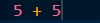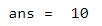HomeBlogArtificial IntelligenceTop 5 Types of Functions in MATLAB 

# Top 5 Types of Functions in MATLAB 

Last updated:
4th Oct, 2022
Views
7 MinsView All## Introduction

MATLAB (MATrix LABoratory) is a programming language developed by MathWorks in 1984. The purpose of writing MATLAB is to provide easy access to matrix software, which was developed by LINPACK (Linear System Package) and EISPACK (Eigen System Package) projects. It is a high-level programming language for numerical computation and programming.

## Top Machine Learning and AI Courses Online

 Master of Science in Machine Learning & AI from LJMU Executive Post Graduate Programme in Machine Learning & AI from IIITB Advanced Certificate Programme in Machine Learning & NLP from IIITB Advanced Certificate Programme in Machine Learning & Deep Learning from IIITB Executive Post Graduate Program in Data Science & Machine Learning from University of Maryland To Explore all our certification courses on AI & ML, kindly visit our page below. Machine Learning Certification

MATLAB is a programming language environment with features like data structure, built-in editing, and debugging tools. It consists of built-in easy to use graphics commands to display results immediately available. It has various built-in commands and math functions that help in mathematical calculation and performing numerical methods.

## Trending Machine Learning Skills

 AI Courses Tableau Certification Natural Language Processing Deep Learning AI

Enrol for the Machine Learning Course from the World’s top Universities. Earn Masters, Executive PGP, or Advanced Certificate Programs to fast-track your career.

## Features

Following are the features of MATLAB –

• It is a high-level programming language used to solve various numerical problems within seconds.
• It has a vast library of mathematical functions, operations, linear algebra, etc.
• Its interface provides tools to maximize performance and improve code quality.
• It consists of a built-in graphical interface for building applications.

## Syntax

MATLAB runs a command prompt tool to execute its output.

For example:

Type the below expression in the command prompt:Type Ctrl+E and the answer will be returned as:## Functions

Functions in the program mean that it accepts inputs and return outputs. Both scripts and functions allow the reuse of commands by storing them into the program files. It provides flexibility because users can pass the input values and return output values. It runs faster as compared to others because it does not store temporary variables.

Functions must be defined within a program file but not at the command line. Following syntax is used to define functions.

function [y1,…,yN] = myfun(x1,…,xM)

Where y1,…,yN are outputs, x1,…,xM are inputs, and

myfun is the function name

Following are some basic rules to be followed for valid function names and saving the functions:

• Valid function names should start from alphabets, then contain a number, underscores.
• Name of the file must match with the first function name in the file.
• The script file name should be different from the function in the file.
• The end keyword is used to indicate the end of the function.

## Types of Functions

Following are the various types of functions –

### 1. Anonymous Functions

An anonymous function is like an inline function that is defined within a single MATLAB statement. It consists of a single MATLAB expression. This function accepts multiple inputs and returns one output. The function that is not stored in a program file. This program file is associated with a variable whose data type is function_handle.

The basic syntax is

function_name = @ (variable_name) matlab_expression;

Where function_name is the name of the Anonymous function. Variable_name is the name of a variable. matlab_expression is a mathematical expression.

### 2. Local functions

MATLAB program files contain a code of multiple functions. The first function in the function file is called as the main function. This main function in one file can be visible to other functions of another file, i.e. the user can call it from the command line. Local functions are defined after the main function and can be visible to other functions in the same file.

For example, create a function file named mystatastic.m that contains a main function, mystatastic, and two local functions, mymeanf and mylocalf.

function [avg, med] = mystatastic(x)

n = length(x);

avg = mymeanf(x,n);

med = mylocalf(x,n);

end

function a = mymeanf(v,n)

% MYMEANF Example of a local function.

a = sum(v)/n;

end

function m = mylocalf(v,n)

% MYLOCALF Another example of a local function.

w = sort(v);

if rem(n,2) == 1

m = w((n + 1)/2);

else

m = (w(n/2) + w(n/2 + 1))/2;

end

end

The local functions mymeanf and mylocalf calculate the average and median of the input list. The main function mystatastic determines the length of the list n and passes it to the local functions.

### 3. Nested functions

A function within a parent function is called a nested function. It can be defined as functions within the body of another function.

Following is the syntax of nested function:

function x = A(p1, p2)

B (p2)

function y = B (p3)

…

end

end

For example, the function with name parentf contains the function nestedf

function parentf

disp(‘This is the parent function’)

nestedf

function nestedf

disp(‘This is the nested function’)

end

end

The advantage of nested functions is that they can access and modify variables that are defined in parent functions.

### 4. Private functions

Private function is useful when the user wants to limit the scope of a function. The function makes the subfolder under it, and it will be available only to the subfolder functions. This subfolder is named as private.

For example, create a subfolder with the name private. Within the private folder, create a file with the name examplefile.m.

function findme

% FINDME An example of a private function.

disp (‘You found the private function’)

Change to the folder that contains the private folder and creates a file named visible.m

function visible

findme

Change your current folder to any location and call the visible function.

Visible

You found the private function.

### 5. Global variable

A variable that is declared as global in all the functions is called a global variable. It can be shared with one or more functions. To declare the global variable at the base workspace, declare the variable at the command line. The ‘global’ word should be mentioned before the variable which we are declaring as a global variable.

Following is the syntax of the global variable:

global var1 … varN

For example, create a function file with name avg.m and follow the below code:

function avgr = avg(nums)

global ALL

avgr = sum(nums)/ALL;

end

Now, create a script file and follow the below code:

global ALL;

ALL = 10;

n = [34, 45, 25, 45, 33, 19, 40, 34, 38, 42];

av = average(n)

The following result will be displayed:

av = 35.500

## Popular AI and ML Blogs & Free Courses

 IoT: History, Present & Future Machine Learning Tutorial: Learn ML What is Algorithm? Simple & Easy Robotics Engineer Salary in India : All Roles A Day in the Life of a Machine Learning Engineer: What do they do? What is IoT (Internet of Things) Permutation vs Combination: Difference between Permutation and Combination Top 7 Trends in Artificial Intelligence & Machine Learning Machine Learning with R: Everything You Need to Know AI & ML Free Courses Introduction to NLP Fundamentals of Deep Learning of Neural Networks Linear Regression: Step by Step Guide Artificial Intelligence in the Real World Introduction to Tableau Case Study using Python, SQL and Tableau

## Conclusion

MATLAB functions can be used as an integral part of programming language. They can be assessed globally by using global variables and can be used privately by using private functions. It can fulfill all the requirements of an organization because of its numerous unique features.

If you’re interested to learn more about MATLAB, machine learning, and its relevant topics, check out IIIT-B & upGrad’s PG Diploma in Machine Learning & AI which is designed for working professionals and offers 450+ hours of rigorous training, 30+ case studies & assignments, IIIT-B Alumni status, 5+ practical hands-on capstone projects & job assistance with top firms.Blog Author
Director of Engineering @ upGrad. Motivated to leverage technology to solve problems. Seasoned leader for startups and fast moving orgs. Working on solving problems of scale and long term technology strategy.
Get Free Consultation

Select CourseSelectBy clicking 'Submit' you Agree to

#### Our Popular Machine Learning Course

1Why is MATLAB useful?

MATLAB is one of the most well-known fourth-generation programming languages and is increasingly used to develop advanced AI and machine learning applications. MATLAB is very useful for performing complex mathematical deductions, analysis, design, and optimization of both mathematical and structural models. It offers a high level of speed, precision, and accuracy for complex calculations involving different kinds of algorithms. Besides, MATLAB allows us to analyze different types of data sources like databases and files, which makes it especially suitable for data science projects. And it also supports different techniques of visualization, simulation of data prototypes and models.

2How can you learn MATLAB?

Since MATLAB is an object-oriented programming language, it will be great if you already have some basic familiarity with the concepts of object-oriented programming. These concepts will help you understand and relate to MATLAB quickly. Next, you will also find it helpful to know how MATLAB develops algorithms and sequences codes. While it is not mandatory for you to understand these concepts before you start learning MATLAB, knowing these can easily enhance your efficiency in writing codes using this programming language. However, you must be familiar with the fundamentals of advanced mathematics, which form the basis of MATLAB's operations.